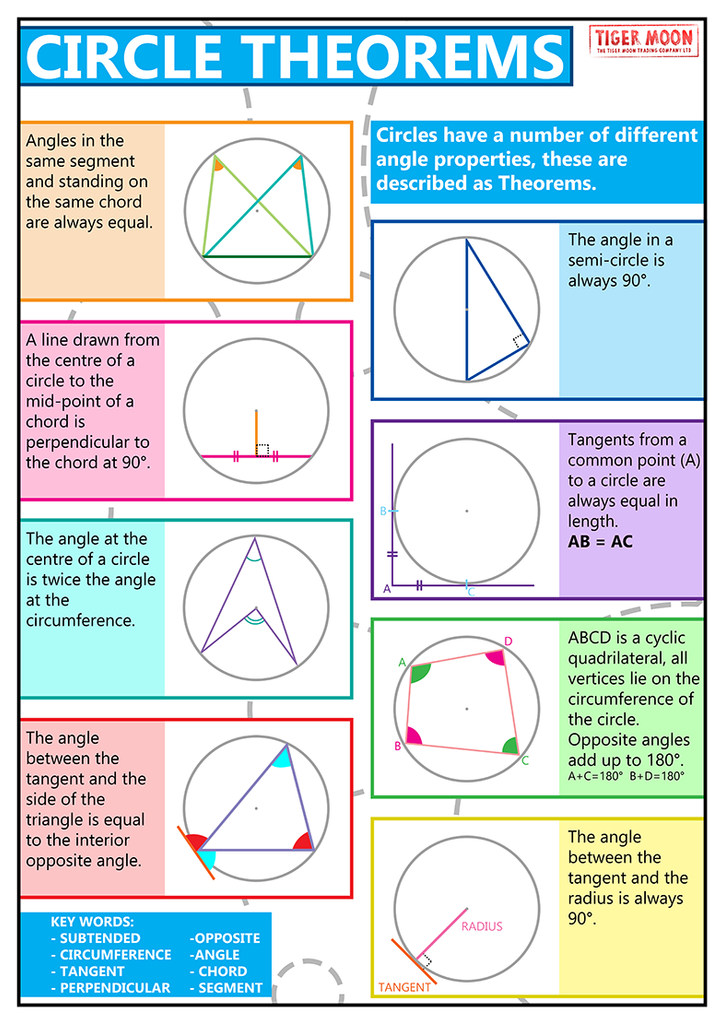# Geometry

the study of shape

Regular Pentagons
& the Golden Ratio

All you need to know about Isosceles Triangles

Reference:
List of polygons
Euler's Formula

The Circle (Simple Wiki).
Measurement of a Circle: a treatise of three propositions by Archimedes.
Equation of a Circle
More on Equation of a Circle
Even more on Equation of a Circle
Circle Theorems
Inscribed Angle Theorem
Intersecting Chords Theorem
If $\left({x}_{1},{y}_{1}\right)$ and $\left({x}_{2},{y}_{2}\right)$ are the co-ordinates of the end of the diameter of a circle, then $\left(x-{x}_{1}\right)\left(x-{x}_{2}\right)+\left(y-{y}_{1}\right)\left(y-{y}_{2}\right)=0$|